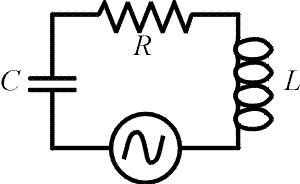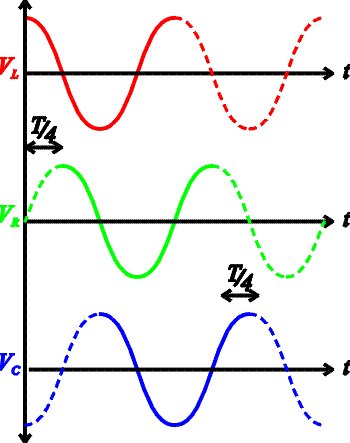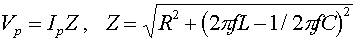LRC circuitsHere we consider circuits with an AC power supply and all three fundamental elements, a resistor, an inductor and a capacitor. The same current flows through each element at every instant in time. The voltages across the four elements must sum to zero when travelling around the loop at any instant as well. Although the voltages across all the elements rise and fall with the same frequency, they do not all have the same phase. When two signals have the same frequencies but different phases, the sine waves representing the signals begin oscillations at different times. There is an offset of the patterns. For instance if we say one signal is 90 degrees behind another, that would mean that the sine wave would be displaced by one fourth of a period.In the figure on the right, the voltage drop across each element is shown as a function of time. The green line, which is the voltage drop across the resistor, also demonstrates the phase of the current. One can see that VL preceeds the current by one fourth of a period. This is because the inductors voltage drop is largest as the current is rising since it is proportional to DI/Dt. The opposite is true of the voltage across the capacitor Vc as it keeps rising until the current is no longer forward. This is because it is proportional to the charge which grows as long as the current is positive. Thus VL and Vc are 180 degrees out of phase, which means they simply have opposite signs. The impedance is the ratio of the peak voltage of the source to the peak current. This impedance depends on the frequency of the source as well as the values of L, C and R. The impedance Z (derivation in next page) can be thought of as the AC analog to resistance.The impedance is at a minimum when the reactance of the inductor, XL = 2pfL,is canceled by the reactance of the capacitor, XC = 1/(2pfC). This is the same resonant condition as discussed for LC circuits previously.